# ggExtra - Add marginal histograms to ggplot2, and more ggplot2 enhancements

`ggExtra` is a collection of functions and layers to enhance ggplot2. The main function is `ggMarginal`, which can be used to add marginal histograms/boxplots/density plots to ggplot2 scatterplots. You can view a live interactive demo to see some of its functionality.

Most other functions/layers are quite simple but are useful because they are fairly common ggplot2 operations that are a bit verbose.

This is an instructional document, but I also wrote a blog post about the reasoning behind and development of this package.

Note: it was brought to my attention that several years ago there was a different package called `ggExtra`, by Baptiste (the author of `gridExtra`). That old `ggExtra` package was deleted in 2011 (two years before I even knew what R is!), and this package has nothing to do with the old one.

## Installation

`ggExtra` is available through both CRAN and GitHub.

To install the CRAN version:

``install.packages("ggExtra")``

To install the latest development version from GitHub:

``````install.packages("devtools")
devtools::install_github("daattali/ggExtra")``````

`ggExtra` comes with an addin for `ggMarginal()`, which lets you interactively add marginal plots to a scatter plot. To use it, simply highlight the code for a ggplot2 plot in your script, and select ggplot2 Marginal Plots from the RStudio Addins menu. Alternatively, you can call the addin directly by calling `ggMarginalGadget(plot)` with a ggplot2 plot.

## Usage

We’ll first load the package and ggplot2, and then see how all the functions work.

``````suppressPackageStartupMessages({
library("ggExtra")
library("ggplot2")
})``````

## `ggMarginal` - Add marginal histograms/boxplots/density plots to ggplot2 scatterplots

You need to have the `grid` and `gtable` packages installed for this function.

This function is meant to work as an easy drop-in solution for adding marginal density plots/histograms/boxplots to a ggplot2 scatterplot. You can either pass it a ready ggplot2 scatterplot and it will add the marginal plots, or you can just tell it what dataset and variables to use and it will generate the scatterplot plus the marginal plots.

As a simple first example, let’s create a dataset with 500 points where the x values are normally distributed and the y values are uniformly distributed, and plot a simple ggplot2 scatterplot.

``````set.seed(30)
df1 <- data.frame(x = rnorm(500, 50, 10), y = runif(500, 0, 50))
(p1 <- ggplot(df1, aes(x, y)) + geom_point() + theme_bw())``````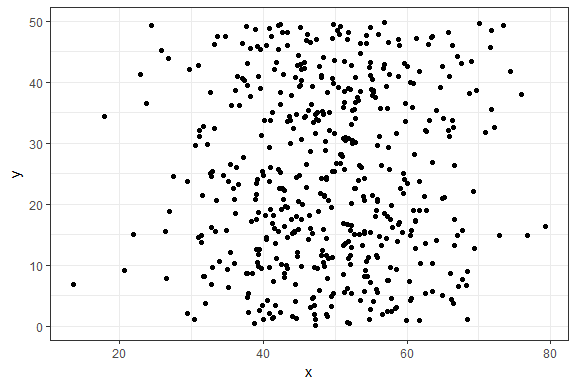Ok, now let’s add marginal density plots.

``ggMarginal(p1)``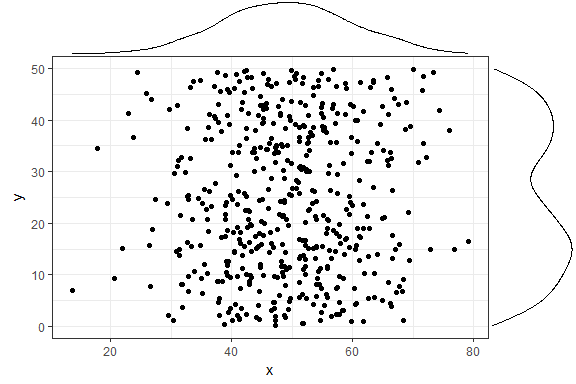That was easy. Notice how the syntax is not following the standard ggplot2 syntax - you don’t “add” a ggMarginal layer with `p1 + ggMarginal()`, but rather ggMarginal takes the object as an argument and returns a different object `ggMarginal(p1)`. This means that you can use magrittr pipes, for example `p1 %>% ggMarginal`.

Let’s make the text a bit larger to make it easier to see.

``ggMarginal(p1 + theme_bw(30) + ylab("Two\nlines"))``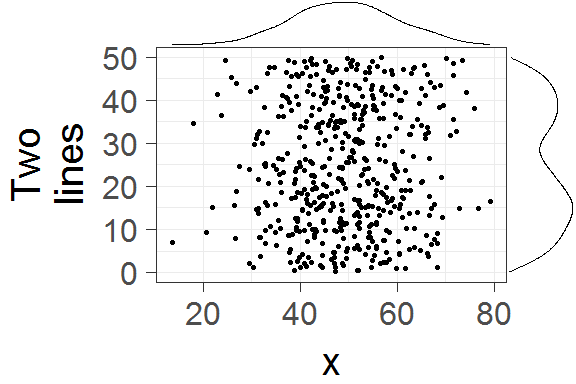Notice how the marginal plots occupy the correct space, and even when the main plot’s points are pushed to the right because of larger text or longer axis labels, the marginal plots automatically adjust.

You can also show histograms instead.

``ggMarginal(p1, type = "histogram")``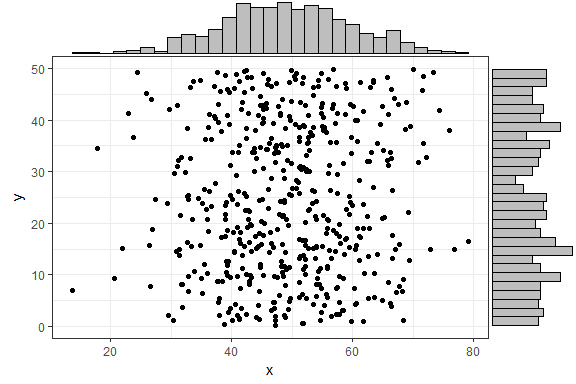There are several more parameters, here is an example with a few more being used. Note that you can use any parameters that the `geom_XXX` layers accept, and they will be passed to those layers, such as `col` and `fill` in the following example.

``````ggMarginal(p1, margins = "x", size = 2, type = "histogram",
col = "blue", fill = "orange")``````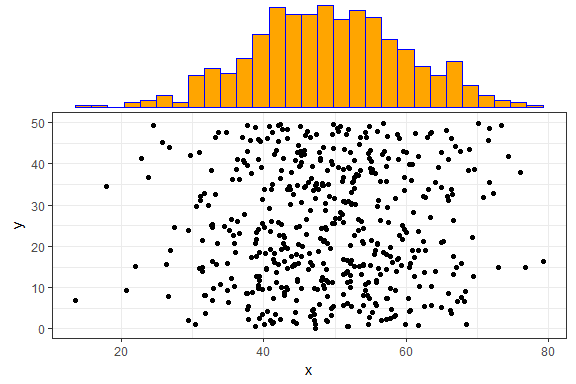In the above example, `size = 2` means that the main scatterplot should occupy twice as much height/width as the margin plots (default is 5). The `col` and `fill` parameters are simply passed to the ggplot layer for both margin plots.

If you want to specify some parameter for only one of the marginal plots, you can use the `xparams` or `yparams` parameters, like this:

``ggMarginal(p1, type = "histogram", xparams = list(binwidth = 1, fill = "orange"))``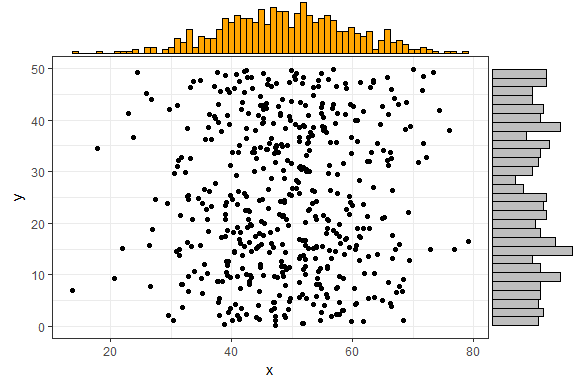You don’t have to supply a ggplot2 scatterplot, you can also just tell ggMarginal what dataset and variables to use, but of course this way you lose the ability to customize the main plot (change text/font/theme/etc).

``ggMarginal(data = mtcars, x = "wt", y = "mpg")``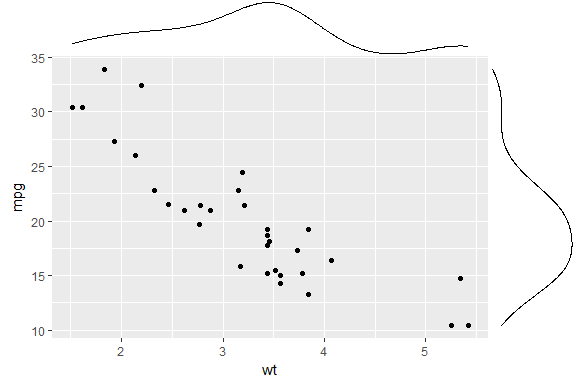Last but not least - you can also save the output from `ggMarginal` and display it later. (This may sound trivial, but it was not an easy problem to solve - see this discussion).

``````p <- ggMarginal(p1)
p``````For more information, see `?ggExtra::ggMarginal`.

## `removeGrid` - Remove grid lines from ggplot2

This is just a convenience function to save a bit of typing and memorization. Minor grid lines are always removed, and the major x or y grid lines can be removed as well (default is to remove both).

`removeGridX` is a shortcut for `removeGrid(x = TRUE, y = FALSE)`, and `removeGridY` is similarly a shortcut for… .

``````df2 <- data.frame(x = 1:50, y = 1:50)
p2 <- ggplot2::ggplot(df2, ggplot2::aes(x, y)) + ggplot2::geom_point()
p2 + removeGrid()``````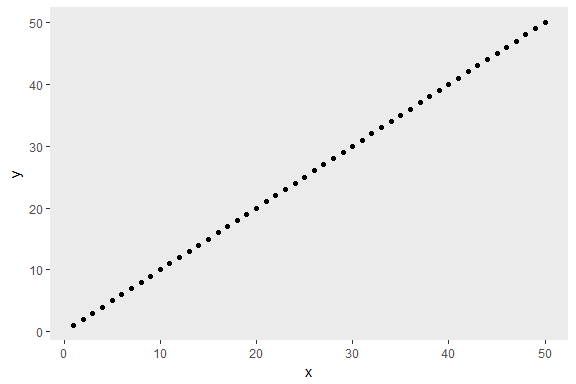For more information, see `?ggExtra::removeGrid`.

## `rotateTextX` - Rotate x axis labels

Often times it is useful to rotate the x axis labels to be vertical if there are too many labels and they overlap. This function accomplishes that and ensures the labels are horizontally centered relative to the tick line.

``````df3 <- data.frame(x = paste("Letter", LETTERS, sep = "_"),
y = seq_along(LETTERS))
p3 <- ggplot2::ggplot(df3, ggplot2::aes(x, y)) + ggplot2::geom_point()
p3 + rotateTextX()``````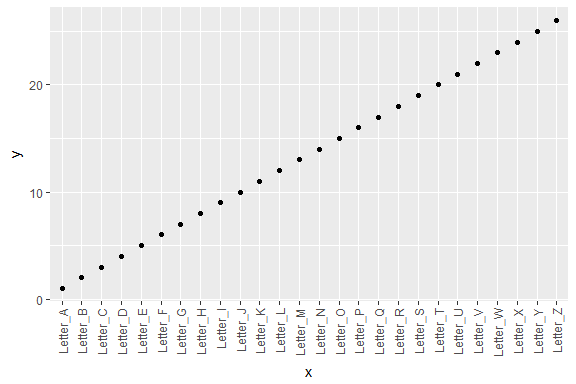For more information, see `?ggExtra::rotateTextX`.

## `plotCount` - Plot count data with ggplot2

This is a convenience function to quickly plot a bar plot of count (frequency) data. The input must be either a frequency table (obtained with `base::table`) or a data.frame with 2 columns where the first column contains the values and the second column contains the counts.

An example using a table:

``plotCount(table(infert\$education))``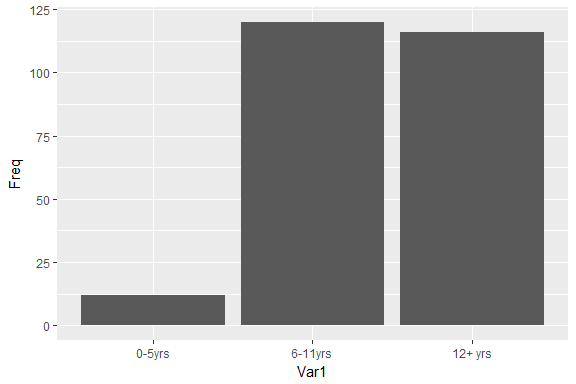An example using a data.frame:

``````df4 <- data.frame("vehicle" = c("bicycle", "car", "unicycle", "Boeing747"),
"NumWheels" = c(2, 4, 1, 16))
plotCount(df4) + removeGridX()``````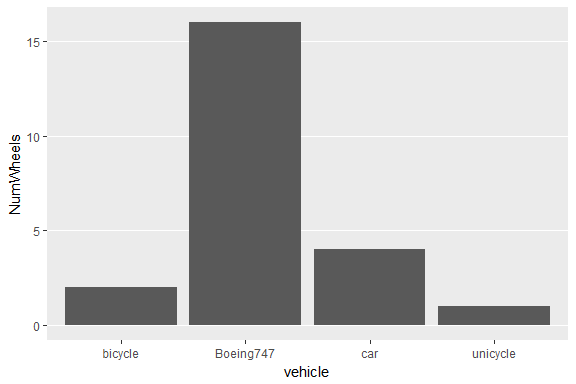For more information, see `?ggExtra::plotCount`.